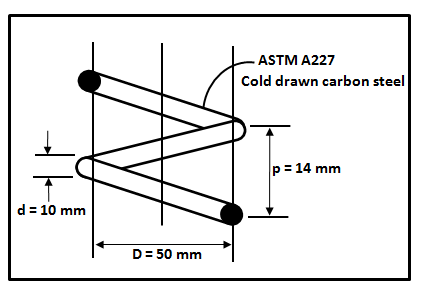# If a helical coil spring with known material and geometrical diametrical as shown below is...

## Question:

If a helical coil spring with known material and geometrical diametrical as shown below is compressed solid, would you expect it to return to its original free-length when the force is removed?## Spring Force:

Spring force is defined as the force that is responsible for stretching the spring or compressing the spring. The Spring force can be obtained by using Hooke's law. The spring force depends on the modulus of elasticity of material by which the spring is made up off.

Given data

• The pitch of the spring is: {eq}p = 14\;{\rm{mm}}{/eq}
• The diameter of the spring wire is: {eq}d = 10\;{\rm{mm}}{/eq}
• The diameter of the effective spring is: {eq}D = 50\;{\rm{mm}}{/eq}
• The spring is ASTM A227 having modulus of elasticity is: {eq}G = 79.289\;{\rm{GPa}}{/eq}

The expression to calculate the maximum bearable torque is given as,

{eq}\tau = \dfrac{{8FD}}{{\pi {d^3}}}\left( {1 + \dfrac{1}{{2c}}} \right){/eq}

Here, {eq}F{/eq} is the force applied on spring,

Calculate the magnitude of force.

{eq}F = \dfrac{{G{d^4}\delta }}{{64n{{\left( {\dfrac{D}{2}} \right)}^3}}}{/eq}

Here, {eq}\delta {/eq} is deflection and {eq}n{/eq} is the number of turns.

Calculate the value of ratio of deflection and number of turns,

{eq}\begin{align*} \dfrac{\delta }{n} &= p - d\\ \dfrac{\delta }{n} &= 14 - 10\\ \dfrac{\delta }{n} &= 4\;{\rm{mm}} \end{align*}{/eq}

Substitute the value in the above expression of force,

{eq}\begin{align*} F& = \dfrac{{79.289 \times {{10}^3} \times {{10}^4} \times 4}}{{64 \times {{25}^3}}}\\ F& = 3171.56\;{\rm{N}} \end{align*}{/eq}

Substitute the value of force in the expression of torque,

{eq}\begin{align*} \tau & = \dfrac{{8 \times 3171.56 \times 50}}{{3.14 \times {{10}^3}}}\left( {1 + \dfrac{1}{{2\left( {\dfrac{{50}}{{10}}} \right)}}} \right)\\ \tau & = 444.42\;{\rm{N/m}}{{\rm{m}}^{\rm{2}}} \end{align*}{/eq}

Since the maximum torque is {eq}444.42\;{\rm{N/m}}{{\rm{m}}^{\rm{2}}}{/eq} and for the cold storage carbon steel have the range of the bearable torque is {eq}\left( {1180 - 1340} \right)\;{\rm{MPa}}{/eq}.

Therefore, it can be concluded that spring will return back to its original free length.Practice Applying Spring Constant Formulas

from

Chapter 17 / Lesson 11
3.3K

In this lesson, you'll have the chance to practice using the spring constant formula. The lesson includes four problems of medium difficulty involving a variety of real-life applications.